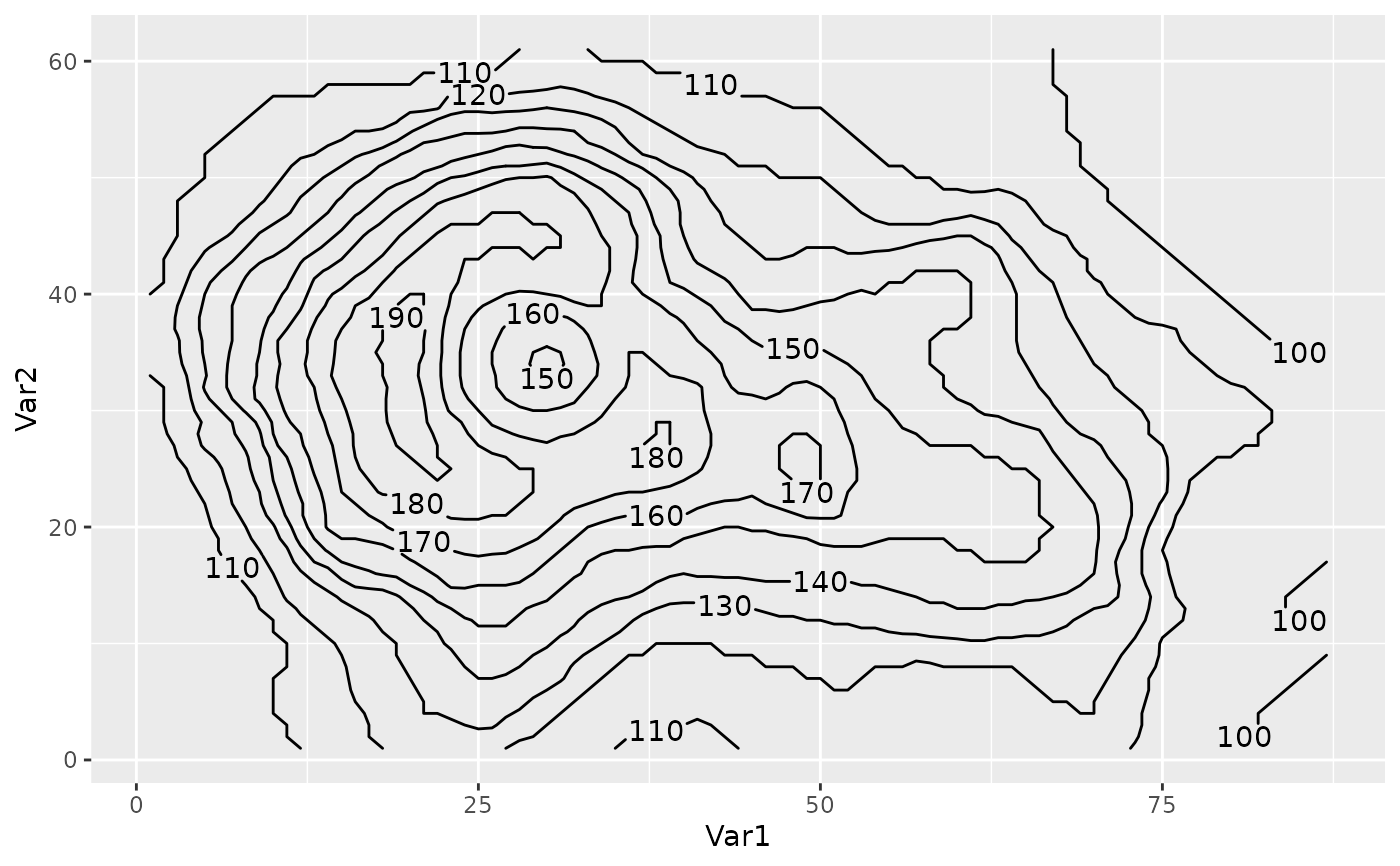Similar to ggplot2::geom_contour but it can label contour lines, accepts accepts a function as the breaks argument and and computes breaks globally instead of per panel.

geom_contour2(
mapping = NULL,
data = NULL,
stat = "contour2",
position = "identity",
...,
lineend = "butt",
linejoin = "round",
linemitre = 1,
breaks = MakeBreaks(),
bins = NULL,
binwidth = NULL,
global.breaks = TRUE,
na.rm = FALSE,
na.fill = FALSE,
skip = 1,
margin = grid::unit(c(1, 1, 1, 1), "pt"),
label.placer = label_placer_flattest(),
show.legend = NA,
inherit.aes = TRUE
)

stat_contour2(
mapping = NULL,
data = NULL,
geom = "contour2",
position = "identity",
...,
breaks = MakeBreaks(),
bins = NULL,
binwidth = NULL,
kriging = FALSE,
global.breaks = TRUE,
na.rm = FALSE,
na.fill = FALSE,
show.legend = NA,
inherit.aes = TRUE
)

## Arguments

mapping

Set of aesthetic mappings created by aes(). If specified and inherit.aes = TRUE (the default), it is combined with the default mapping at the top level of the plot. You must supply mapping if there is no plot mapping.

data

The data to be displayed in this layer. There are three options:

If NULL, the default, the data is inherited from the plot data as specified in the call to ggplot().

A data.frame, or other object, will override the plot data. All objects will be fortified to produce a data frame. See fortify() for which variables will be created.

A function will be called with a single argument, the plot data. The return value must be a data.frame, and will be used as the layer data. A function can be created from a formula (e.g. ~ head(.x, 10)).

stat

The statistical transformation to use on the data for this layer, either as a ggproto Geom subclass or as a string naming the stat stripped of the stat_ prefix (e.g. "count" rather than "stat_count")

position

Position adjustment, either as a string naming the adjustment (e.g. "jitter" to use position_jitter), or the result of a call to a position adjustment function. Use the latter if you need to change the settings of the adjustment.

...

Other arguments passed on to layer(). These are often aesthetics, used to set an aesthetic to a fixed value, like colour = "red" or size = 3. They may also be parameters to the paired geom/stat.

lineend

Line end style (round, butt, square).

linejoin

Line join style (round, mitre, bevel).

linemitre

Line mitre limit (number greater than 1).

breaks

One of:

• A numeric vector of breaks

• A function that takes the range of the data and binwidth as input and returns breaks as output

bins

Number of evenly spaced breaks.

binwidth

Distance between breaks.

global.breaks

Logical indicating whether breaks should be computed for the whole data or for each grouping.

na.rm

If FALSE, the default, missing values are removed with a warning. If TRUE, missing values are silently removed.

na.fill

How to fill missing values.

• FALSE for letting the computation fail with no interpolation

• TRUE for imputing missing values with Impute2D

• A numeric value for constant imputation

• A function that takes a vector and returns a numeric (e.g. mean)

skip

number of contours to skip for labelling (e.g. skip = 1 will skip 1 contour line between labels).

margin

the margin around labels around which contour lines are clipped to avoid overlapping.

label.placer

a label placer function. See label_placer_flattest().

show.legend

logical. Should this layer be included in the legends? NA, the default, includes if any aesthetics are mapped. FALSE never includes, and TRUE always includes. It can also be a named logical vector to finely select the aesthetics to display.

inherit.aes

If FALSE, overrides the default aesthetics, rather than combining with them. This is most useful for helper functions that define both data and aesthetics and shouldn't inherit behaviour from the default plot specification, e.g. borders().

geom

The geometric object to use to display the data, either as a ggproto Geom subclass or as a string naming the geom stripped of the geom_ prefix (e.g. "point" rather than "geom_point")

kriging

Logical indicating whether to perform ordinary kriging before contouring. Use this if you want to use contours with irregularly spaced data.

## Aesthetics

geom_contour2 understands the following aesthetics (required aesthetics are in bold):

Aesthetics related to contour lines:

• x

• y

• z

• alpha

• colour

• group

• linetype

• size

• weight

Aesthetics related to labels:

• label

• label_colour

• label_alpha

• label_size

• family

• fontface

## Computed variables

level

height of contour

Other ggplot2 helpers: MakeBreaks(), WrapCircular(), geom_arrow(), geom_contour_fill(), geom_label_contour(), geom_relief(), geom_streamline(), guide_colourstrip(), map_labels, reverselog_trans(), scale_divergent, scale_longitude, stat_na(), stat_subset()

Other ggplot2 helpers: MakeBreaks(), WrapCircular(), geom_arrow(), geom_contour_fill(), geom_label_contour(), geom_relief(), geom_streamline(), guide_colourstrip(), map_labels, reverselog_trans(), scale_divergent, scale_longitude, stat_na(), stat_subset()

## Examples

data.table::setDTthreads(1)

library(ggplot2)

# Breaks can be a function.
ggplot(reshape2::melt(volcano), aes(Var1, Var2)) +
geom_contour2(aes(z = value, color = after_stat(level)),
breaks = AnchorBreaks(130, binwidth = 10))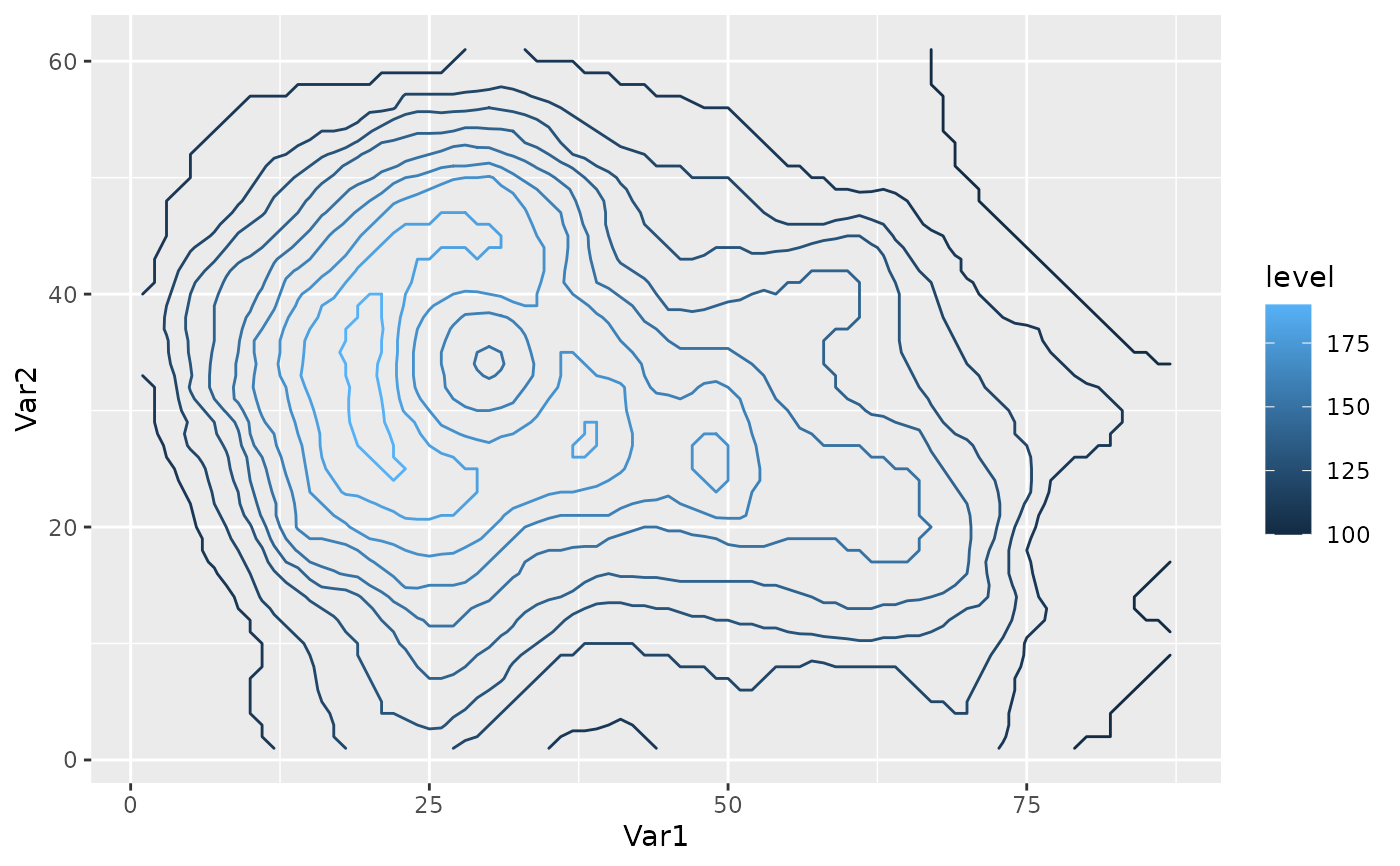# Add labels by supplying the label aes.
ggplot(reshape2::melt(volcano), aes(Var1, Var2)) +
geom_contour2(aes(z = value, label = after_stat(level)))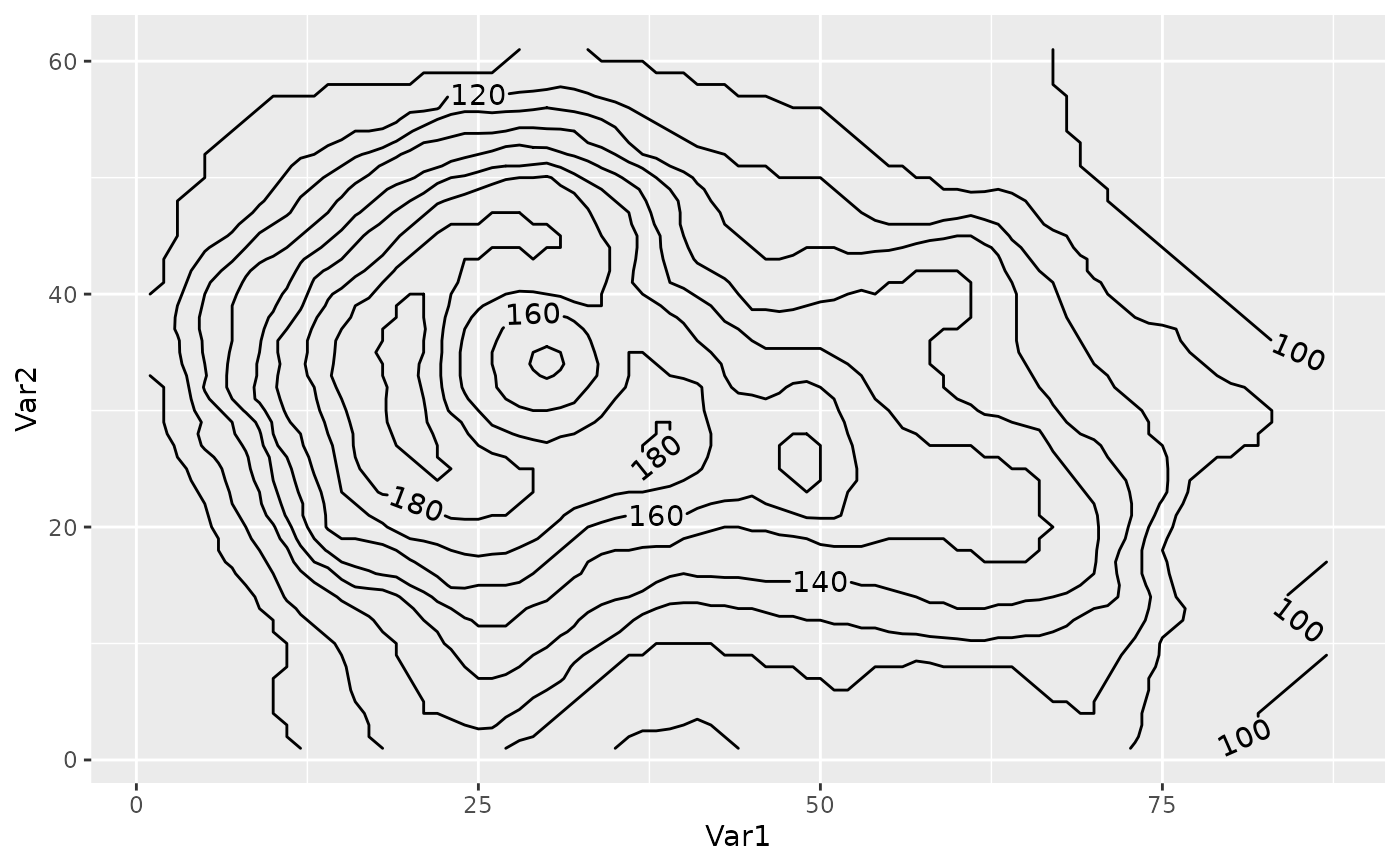ggplot(reshape2::melt(volcano), aes(Var1, Var2)) +
geom_contour2(aes(z = value, label = after_stat(level)),
skip = 0)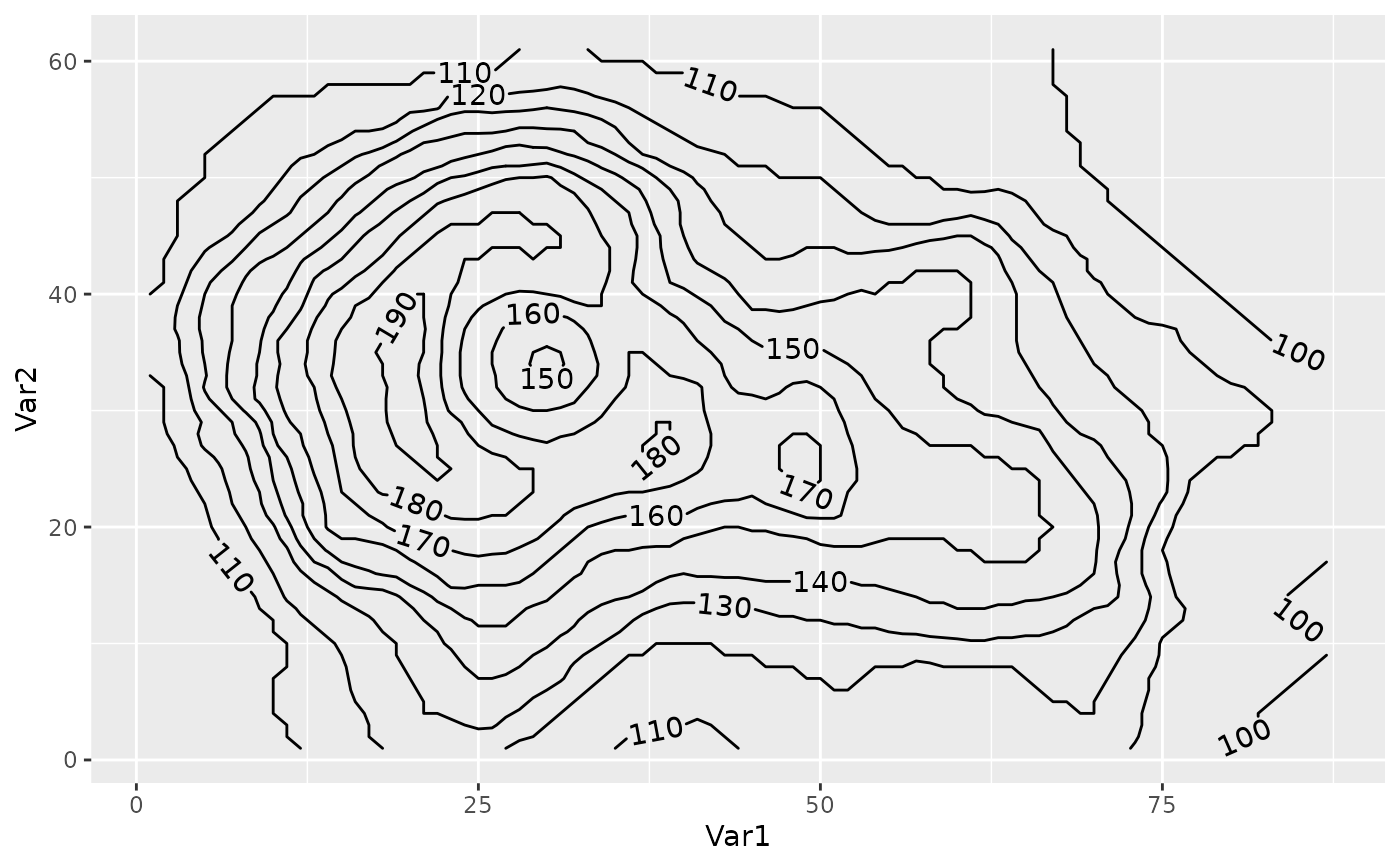# Use label.placer to control where contours are labelled.
ggplot(reshape2::melt(volcano), aes(Var1, Var2)) +
geom_contour2(aes(z = value, label = after_stat(level)),
label.placer = label_placer_n(n = 2))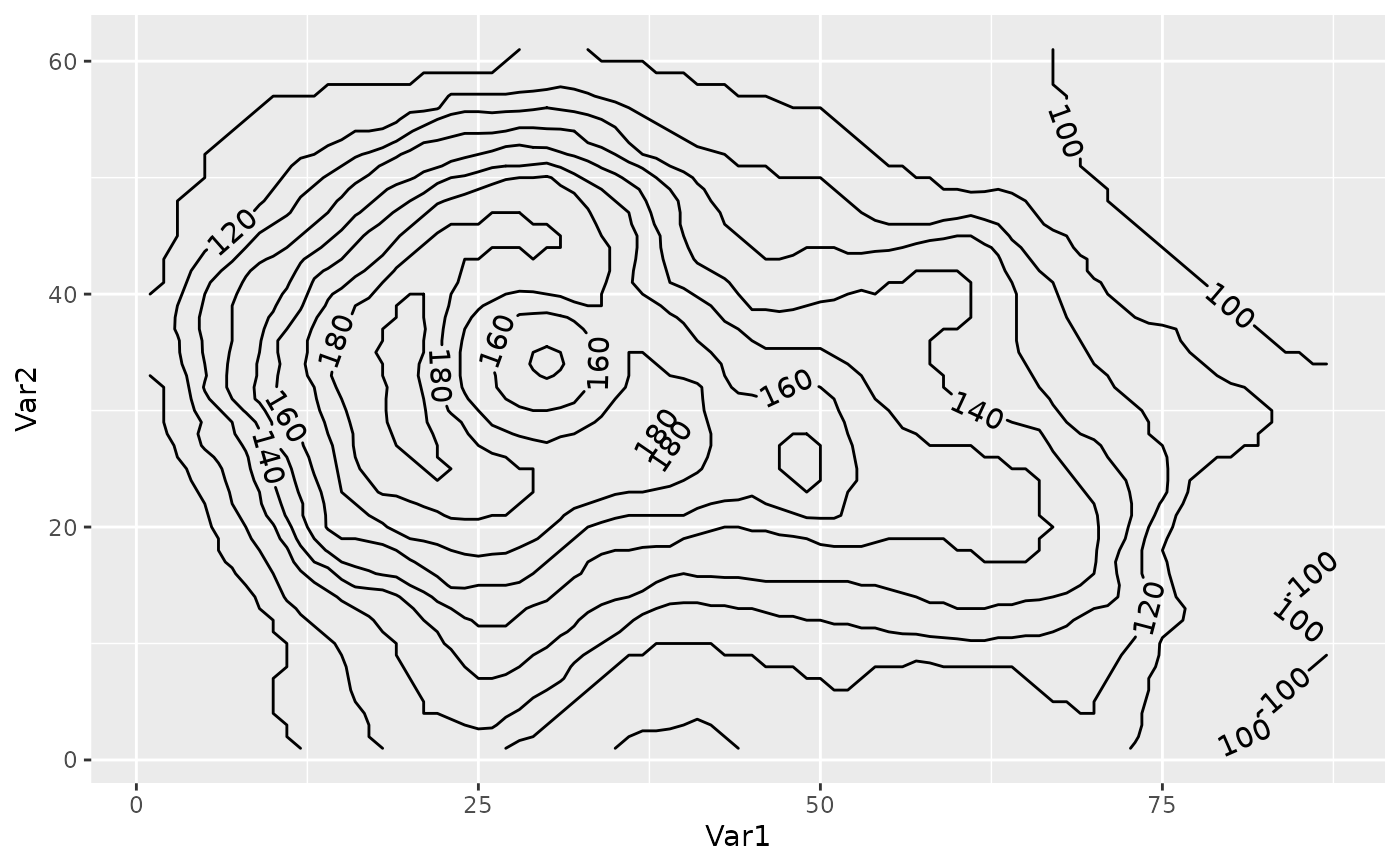# Use the rot_adjuster argument of the placer function to
# control the angle. For example, to fix it to some angle:
ggplot(reshape2::melt(volcano), aes(Var1, Var2)) +
geom_contour2(aes(z = value, label = after_stat(level)),
skip = 0,